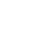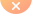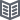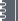xshellcn > Xshell专栏 > 掌握这几个XShell使用技巧，提高主机管理效率

# 掌握这几个XShell使用技巧，提高主机管理效率

1. Xshell当前版本为Xshell 7，相比于Xshell 6在功能上也有了优化。
2. 自动复制粘贴

1. 在Vi编辑器中启用小键盘

1. 更改配色方案，提高视觉效果

1、isayme [isayme]

text(bold)=eaeaea

magenta(bold)=ff00ff

text=ffffff

white(bold)=eaeaea

green=00c000

red(bold)=d20000

green(bold)=00ff00

black(bold)=808080

red=c00000

blue=113fcc

black=000000

blue(bold)=0080ff

yellow(bold)=ffff00

cyan(bold)=00ffff

yellow=c0c000

magenta=c000c0

background=222222

white=c0c0c0

cyan=00c0c0

[Names]

count=1

name0=isayme

2、SolarizedDarkModify亮色版

[SolarizedDarkModify]

text=839496

cyan(bold)=00ffff

text(bold)=e9e9e9

magenta=c000c0

green=80ff00

green(bold)=3c5a38

background=042028

cyan=00c0c0

red(bold)=ff0000

yellow=c0c000

magenta(bold)=ff00ff

yellow(bold)=ffff00

red=ff4500

white=c0c0c0

blue(bold)=1e90ff

white(bold)=fdf6e3

black=000000

blue=00bfff

black(bold)=808080

[Names]

name0=SolarizedDarkModify

count=1

3、Solarized Dark暗色版

[Solarized Dark]

text=839496

cyan(bold)=93a1a1

text(bold)=408080

magenta=dd3682

green=859900

green(bold)=586e75

background=042028

cyan=2aa198

red(bold)=cb4b16

yellow=b58900

magenta(bold)=6c71c4

yellow(bold)=657b83

red=808000

white=eee8d5

blue(bold)=8080ff

white(bold)=fdf6e3

black=002b36

blue=268bd2

black(bold)=073642

[Names]

name0=Solarized Dark

count=1热点问题相关新闻